# How to count without duplicates in Excel

Data analysis usually involves large data sets, and at some point, one may need to find out the number of values that appear only once in the dataset. In this case, you will be counting without duplicates. Unique values are values that appear only once in a dataset.

If you are faced with mountains of data, then counting without duplicates might be very arduous. More so, Excel does not have a special formula for counting without duplicates. However, there is always a way out! This tutorial will serve as a guide on how to count unique and distinct values without duplicating them.Take a look at the numbers in the table above; the unique values are not duplicated. They don't appear more than once. Whereas, distinct values are the different numbers in the collection. In the table below, we have separated the unique values from distinct values.## 1. Using the array COUNTIF formula

The COUNTIF function counts the frequency of occurrence of each value within the range. To get the number of the unique values, you have to sum them up. You can do this efficiently by combining SUM and COUNTIF functions. A combo of two functions can count unique values without duplication.

Below is the syntax:

=SUM(IF(COUNTIF(data, data)=1,1,0)).

The formula contains three separate functions – SUM, IF, and COUNTIF.

COUNTIF function" counts how many times a particular number appears within the range.

"IF function" analysis the results returned by the "COUNTIF" function. It maintains the 1's for unique values and replaces other values with "0."

Note: Always press Ctrl + Shift + Enter when entering your array formula.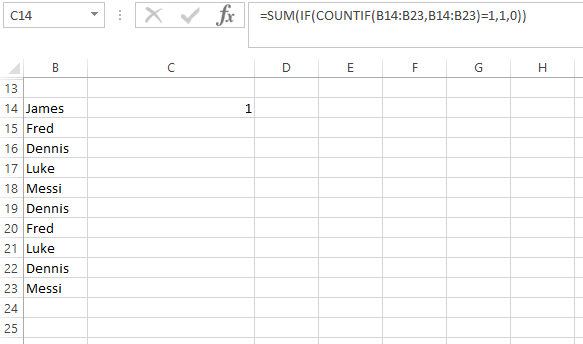CTRL+SHIFT+ENTER allow excel to recognize the formula as an array function.  unique value= =SUM(IF(COUNTIF(A2:A10, A2:A10)=1,1,0))

Alternatively, you can use the SUMPRODUCT formula to avoid the use of CTRL+SHIFT+ENTER.

Below is the syntax:

=SUMPRODUCT(1/COUNTIF(range, criteria)).

In the example below, we have a list of items with duplicates.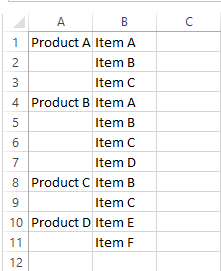If we use the SUM formula to count the total number of items, there will be duplicates. To exclude the duplicates, you have to follow these steps.

Step 1: Go to cell D1 and enter this formula “=SUMPRODUCT(1/COUNTIF( B1:B11,B1:B11)). B1:B11 is the array range you want to count the total number of unique values in the list.Step 2: Press enter and the results will be displayed in cell D1. From the displayed results (6) we can see there are no duplicates.The above formula counts the values of the six items A/B/C/D/E/F.

## 2. Using a combination of SUM, IF, FREQUENCY, MATCH, and ROW Function

If we want to count unique values that exclude all duplicates (that appear in more than one product), use the combination of these functions.

• The sum function allows you to add the values.
• For each true condition of IF function, assign value 1.
• The FREQUENCY function allows you to count the number of values ignoring texts and zeros. In the first occurrence of the distinct value, it returns an equal number to the number of occurrences of that value. It returns zero for the occurrences that have the same value after the first occurrence.
• The MATCH function returns the position of the text value in an array range. The return value acts as the function argument for FREQUENCY.
• The ROW function returns the row reference number.

Example: Enter the following formula to count unique values by excluding all duplicates

=SUM(IF(FREQUENCY(MATCH(B1:B11,B1:B11,0),ROW(B1:B11)-ROW(B1)+1)=1,1))Press enter. The unique value is 3 (Item D/E/F).## 3. Using ISNUMBER Function to count numeric numbers

The function we used earlier counts, both texts and numbers, without duplicating. To count only numerals without duplicating, you have to include ISNUMBER function in the formula for finding unique values.

=SUM(IF(ISNUMBER(A2:A10)*COUNTIF(A2:A10,A2:A10)=1,1,0))

Note: Always press Ctrl + Shift + Enter when entering your array formula.

Meanwhile, the lowdown in this function is that it also counts dates and times.## 4. Using ISTEXT Function to count text values

You can count the number of texts without duplicating by including the ISTEXT function in the array formula as stated below:

=SUM(IF(ISTEXT(A2:A10)*COUNTIF(A2:A10,A2:A10)=1,1,0))

This formula will display the number of unique texts. It excludes errors, blank cells, logical numbers, numbers, etc.

Always press Ctrl + Shift + Enter when entering your array formula.## 5. Using Pivot Table to count text values

Easies way to count the value is by creating the pivot table and using the Count of items

1. Create a pivot table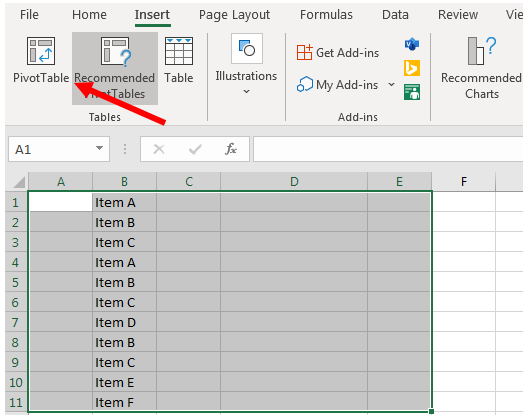2. Use Count of Items3. Output Table## 6. Using A Filter

The Filter method is the simplest approach that allows you to remove duplicates and remain with unique values. You can, therefore, use two tricks to get unique values as discussed below.

### 6.1: Filtering for Unique Values

Steps:

1. Select and make sure the range of cells you want to make unique is in a table.

2. Click on Data then select the Advanced option in the soft & filter group.3. When the Advanced Filter box pops up, you can filter the range of cells or tables by clicking on the Filer the List, in-place option.4. You can also copy the result of the filter to another location by clicking on the Copy to another location option.

5. Enter a cell reference in the Copy To box.6. Alternatively, you can click the Collapse Dialog option to hide the popup window temporarily, select a cell on the worksheet, and then click the Expand option.

7. Finish by checking the Unique Records Only and click OK.### 6.2: Removing The Duplicates

The method only affects the values in the range of cells or tables and not values outside. After removing the duplicates, you will keep the first occurrence of the value in the list, while the identical values will be deleted. Therefore, remember to copy the original range of cells to the table to another worksheet before deleting duplicates.

Steps:

1. Start by selecting the active range of cells in a table.

2. Select the Data Tab and click Remove Duplicates in the Data Tools group.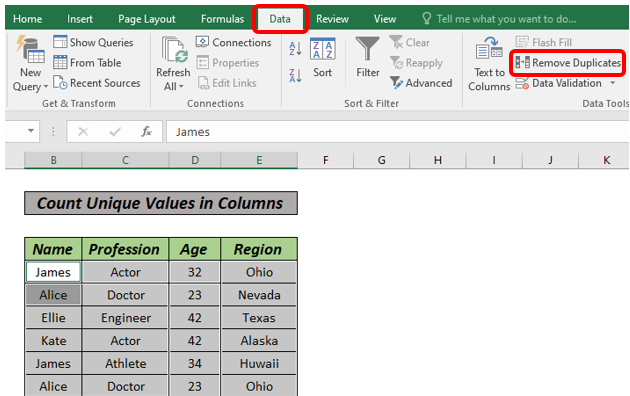3. You can select one or more columns under the Columns face. Alternatively, you can click Select All to select all columns quickly or Unselect All to clear all columns quickly.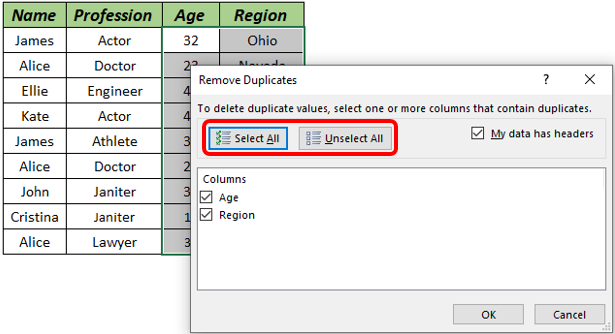4. Click OK and a message will pop up showing how many duplicates you have removed or how many unique values remain. To dismiss the message, click OK.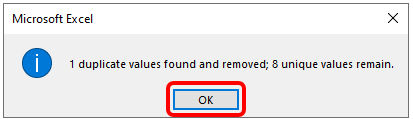5. You can undo the change by clicking Undo or pressing Ctrl + Z

## 7. Using Amazing Excel Tools

Another simple way to count without duplicates in excel is tools like Kutools by the following steps.

1. Select a blank cell to output the result.

2. Click Kutools>Formula>Helper>Formula Helper.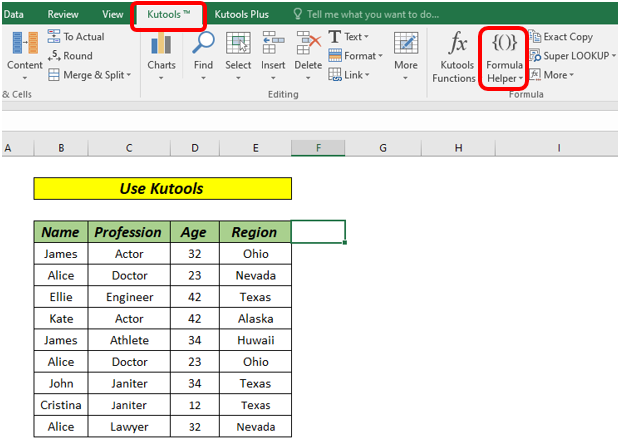3. Do the following steps in the formula helper dialog;

• Check and select Count unique values in the Choose a formula box. You can check the filter box and type some words to filter the formula names.
• Then choose the range in which you want to count unique values in the range box.
• Press the OK button.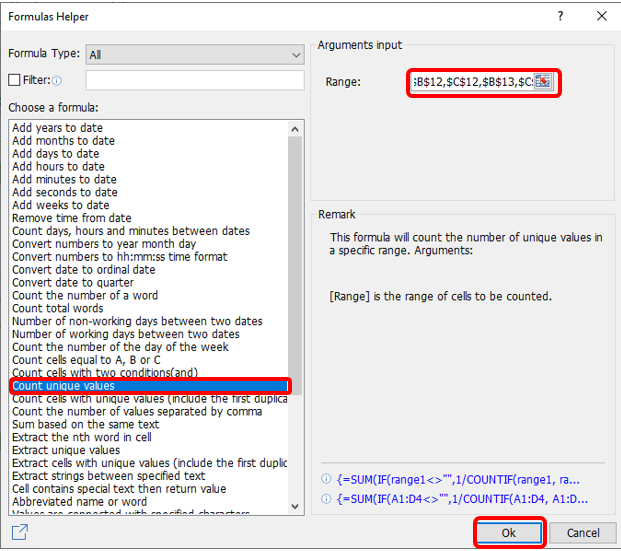## 8. Using A Data Model with A Pivot Table to Count Unique Values

The method applies to newer versions of Microsoft Excel, including Microsoft 365, Excel 2019, Excel 2016, and Excel 2013. To count unique values using this approach, follow these steps:

1. Select any cell in the data and click on the Insert tab in the ribbon.

2. Click on Pivot Table to open a dialogue box.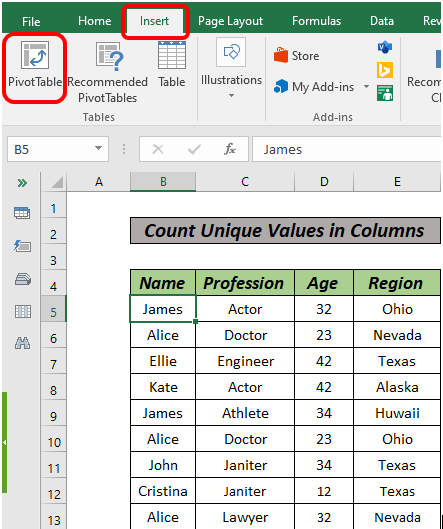3. Select the Add this data to the Data Model at the bottom and click OK.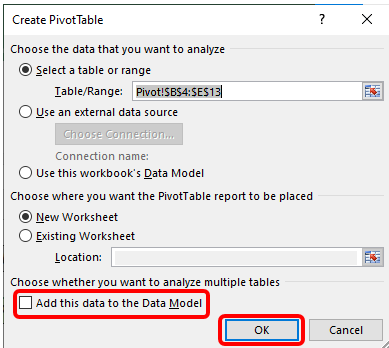4. In the resulting window, drag the Name column into the Name field and the Profession column or any other in the dataset into the Values field.5. Click on the small arrow next to the Count of Profession option in the Pivot Table fields.

6. Select the Value Field Settings at the bottom.7. Finish by selecting the Distinct Count or Unique Count option and click OK.8. You will have your unique values in the Pivot Table.## 9. Using Power Pivot

Power Pivot is also another powerful method you can use to count unique values. However, you will need an enabled Power Pivot tab in your ribbon. That means if your Excel version does not have an already-enabled Power Pivot add-in, you will have to enable it first. Once you have enabled it, you can proceed with the following steps:

1. Go to the Data Model and select the Manage Button.2. This will open a new blank window if it is your first time importing data.

3. Click on Home Tab and select the “Get External Data” option.4. You will see various sources and options to upload data. Since you want to upload simple Excel, select the "From Other Sources" option.

5. Scroll down to the end of a new dialogue box opened, select “Excel File”, and click Next.6. You will need to rename the connection created. For simplicity, you can rename it “Excel”. After that, click on “Browse” to choose an Excel file path.

7. You can click on the “Use the first row as column header” option to make the top column the header. Click on the Next Button to finish.8. You will see the file imported to the Data Model. Click the Finish button.9. After importing all the rows successfully, hit the Close Button.10. With your new worksheet, you will have to create a Pivot Table by clicking on Home and then Pivot Table.11. Click on the small triangle next to it to expand the columns. That is because you already have the data on the first sheet.12. After dragging and dropping all the data in their respective fields, you will get a simple Pivot Table.13. Go back to the Power Pivot window and select the Measure option to create a New Measure.14. Add your desired description name by typing in the box and the suggestions will pop up automatically. Since you want to count unique values, select the Unique Count function.

15. Press the Tab button or use the bracket to select which column you need the unique count for. For example, if you want the unique count for Profession, your formula will be like this;

=DISTINCTCOUNT (Sheet1[Profession]))

16. Select the Number category since you want to count unique values.

17. Change the format to “Whole Number’ and hit OK to create a new column with unique entries.I hope this tutorial is comprehensive. Kindly share with friends & thanks for reading!